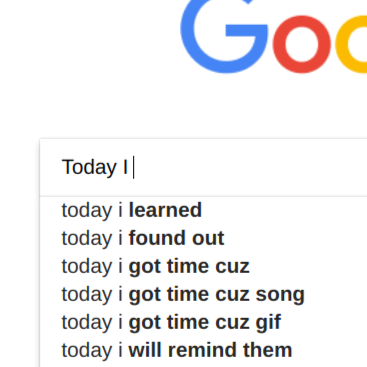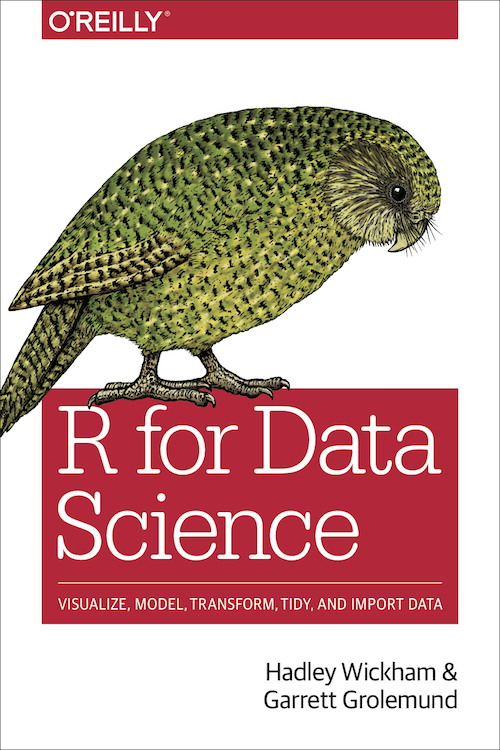# Chunji Wang

#### Vocabulous 0.1: Build Your Own Vocabulary Review Book

Motivation Exactly 11 months ago, I wrote a blog post titled How to Break Vocabulary Bottleneck, in which I talked about how learning new English words has been a challenge for college graduates. I think there are basically three reasons why it has become a challenge: There are no exams, texts, and deadlines anymore as sources of motivation; Exposure to new words is not enough for memorization given the nature of a long tail distribution of words; We are constantly distracted.#### Word Prediction via Ngram Model

If you don’t know what it is, try it out here first! If you just want to see the code, checkout my github. OK, if you tried it out, the concept should be easy for you to grasp. A gram is a unit of text; in our case, a gram is a word. In this application we use trigram – a piece of text with three grams, like “how are you” or “today I meet”.#### Generate My Personal Logo

I generated my personal logo with the following code. It was fun. library(tidyverse) The squares are represented by x,y coordinates, together with id and color: gap <- 0.0 square <- tibble(y = c(0,1,2, 1), x = c(0,1,0,-1)) square2 <- mutate(square, x = x - 1 - gap, id = 2, color = 1) square4 <- mutate(square, x = x + 1 + gap, id = 4, color = 1) square3 <- mutate(square, y = y + 1 + gap, id = 3, color = 2) square1 <- mutate(square, x = x - 2 - 2*gap, y = y + 1 + gap, id = 1, color = 2) square5 <- mutate(square, x = x + 2 + 2*gap, y = y + 1 + gap, id = 5, color = 2) squares <- bind_rows(square1, square2, square3, square4, square5) Logo is rendered through geom_polygon():#### Selected Solutions to Exercises in R for Data Science

R for Data Science (R4DS) is an excellent book about doing data science with R. Here are some solutions I came up with while reading the book. library(tidyverse) library(nycflights13) 3.6.1.6 “Recreate the R code necessary to generate the following graphs”: the last graph ggplot(data = mpg, aes(x = displ, y = hwy)) + geom_point(color = "white", size = 4) + geom_point(aes(color = drv)) 5.3.1.1 “How could you use arrange() to sort all missing values to the start?